# SAT Math Multiple Choice Question 708: Answer and Explanation

### Test Information

Question: 708

3. The functions f, g, and h are defined by the equations f(x) = x2, g(x) = x, and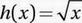. Which of the following must be true?

• A.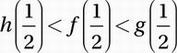• B.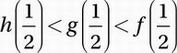• C.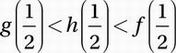• D.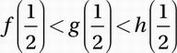Explanation:

D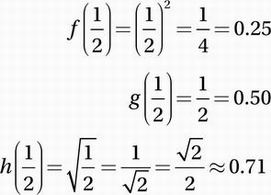Therefore,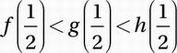.